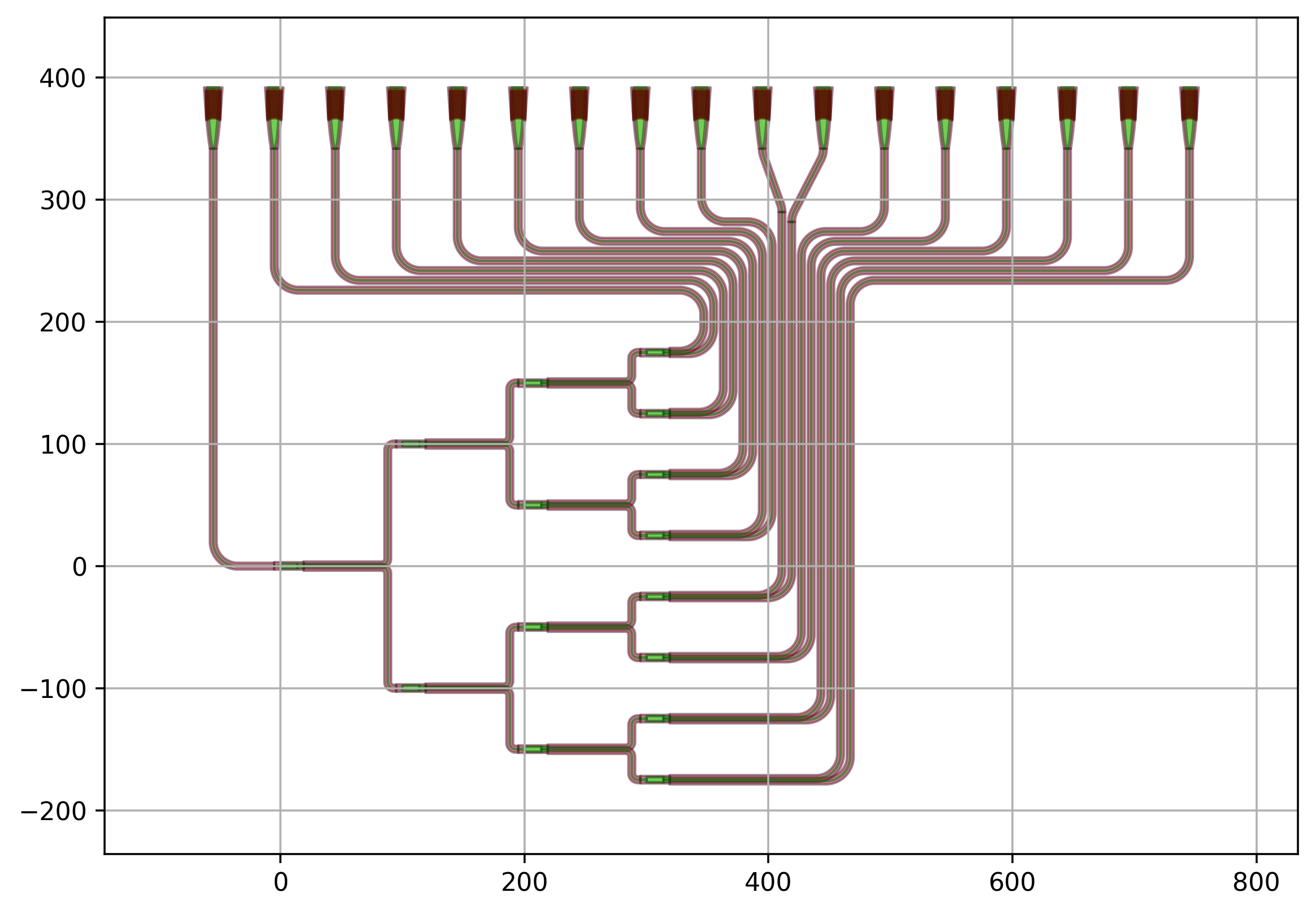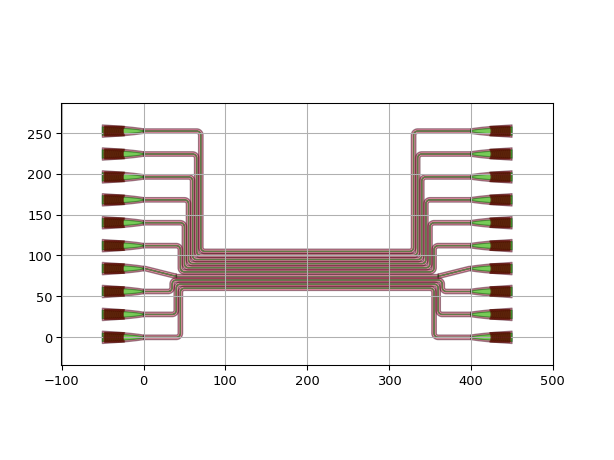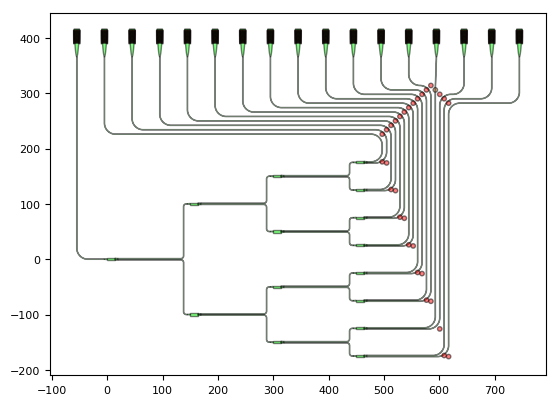# 1. Advanced Routing: Routing to the chip edge¶

## 1.1. Introduction¶

Connecting components is an important part of every integrated photonics design. In Waveguides and waveguide connectors an introduction is given on how to connect two ports with each other using a single connector. This is usually done in the context of a CircuitCell class that uses waveguide connectors to connect two components:

connectors = [("sp_0_0:out1", "sp_1_0:in1", bezier_sbend, {"adiabatic_angle": 1.0}),
("sp_0_0:out2", "sp_1_1:in1", bezier_sbend)]


The list of connectors offers an overview of what is connected and how. We recommend that for any circuit you make, whether simple or complex, you use a list of connectors as it provides a clear overview of your intended connections and a way to customize them.

As documented in Waveguides and waveguide connectors, a list of ready-made connectors is provided that you can use to connect your components with each other. This approach is usually enough for simple connections that don’t need to be customized beyond the parameters of the connector function. Before we get into more complicated examples, let’s warm up with a simple example using simple connectors.

## 1.2. Warm-up¶

Start from the skeleton exercise.py, where all the waveguides overlap.

from si_fab import all as pdk
from ipkiss3 import all as i3
from circuit.circuitcell import CircuitCell
from circuit.connector_functions import manhattan
import numpy as np
grating = pdk.FC_TE_1550()
spacings = np.linspace(0, 10, 5)
child_cells = dict()

spacing = 5.0
place_specs = []
connectors = []

for cnt, sp in enumerate(spacings):
gr_up = "gr_up_{}".format(cnt)
gr_down = "gr_down_{}".format(cnt)
gr_up_port = "{}:out".format(gr_up)
gr_down_port = "{}:out".format(gr_down)
child_cells[gr_up] = grating
child_cells[gr_down] = grating
place_specs.append(i3.Place(gr_up_port, (spacing_x, (-cnt / 2.0 - 0.5) * spacing_y)))
place_specs.append(i3.Place(gr_down_port, (0, (cnt / 2.0 + 0.5) * spacing_y)))
connector = (
gr_up_port,
gr_down_port,
"min_straight": 0.0,
"control_points": [(50, 0.0)]}
)
connectors.append(connector)

cell = CircuitCell(
child_cells=child_cells,
place_specs=place_specs,
connectors=connectors
)

cell.Layout().visualize(annotate=True)Try to fix this design to obtain the following layout of a grating array:Solution

## 1.3. Routing to the chip edge¶

An image tells more than a thousand words. Let’s have a look at the following routed splitter tree.A careful look at the waveguides in this design shows that although they are all part of the same bundle, their path strongly varies. Some waveguides:

• Just have one bend (input waveguide)
• Route around the corner and go up (gratings to the left)
• Route up and have an S-bend at the end (gratings in the middle)
• Route up and to the right (gratings to the right)

Which approach to take depends on the relative positioning of the grating to the output port of the splitter tree. While IPKISS does not have full autorouting capability, it is a very convenient platform to use code to implement a routing heuristic for your circuits that accommodates parametric changes to your device-under-test (splitter tree in this example).

Taking the time to code a routing heuristic has the following advantages:

• Heuristics translate well from one design to the next.
• A routing heuristic can be improved step-by-step.
• A routing heuristic is fully customizable.
• A routing heuristic saves you a lot of work when making small changes to your device-under-test.

In this tutorial we will use examples introduce the coding practices used in the routed splitter tree.

## 1.4. Combine Connectors¶

In the example above we have connectors that use a Manhattan route in the first portion and switch to an S-bend in the second portion. This is the case for the waveguides that are routed to the gratings in the middle. One can use the function combine_connectors to make one connector built from two or more individual sections.

Let’s have a look at the following example. Two grating couplers are connected using an S-bend from the first grating (gc1) up to (100, 100) and a manhattan routing from (100, 100) to the second grating (gc2).

from si_fab import all as pdk
from circuit.circuitcell import CircuitCell
from circuit.connector_functions import manhattan, sbend
from circuit.combine_connectors import combine_connectors
from ipkiss3 import all as i3

gr = pdk.FC_TE_1550()
child_cells = {"gc1": gr,
"gc2": gr}
specs = [i3.Place("gc2", (200, 200)), i3.FlipH("gc2")]
cf = combine_connectors([sbend, manhattan], [(100, 100)])

cell = CircuitCell(child_cells=child_cells,
place_specs=specs,

cell.Layout().visualize()The function combine_connectors creates a new connector from a list of existing ones and a list of intermediary points. The new connector can be used in CircuitCell just like any other connector. In this first example, the bend_radius of all the subsections of the combined connector is set when it’s used in CircuitCell.

Sometimes it is required to set the properties of the connectors on an individual level. This can be done using partials, as demonstrated in the example below where a control point is added to the manhattan section of the combined connector.

from si_fab import all as pdk
from circuit.circuitcell import CircuitCell
from circuit.connector_functions import manhattan, sbend
from circuit.combine_connectors import combine_connectors
from functools import partial
from ipkiss3 import all as i3

gr = pdk.FC_TE_1550()
child_cells = {"gc1": gr,
"gc2": gr}
specs = [i3.Place("gc2", (200, 200)), i3.FlipH("gc2")]

mat = partial(manhattan, control_points=[(150, 150)])
cf = combine_connectors([sbend, mat], [(100, 100)])

cell = CircuitCell(child_cells=child_cells,
place_specs=specs,

cell.Layout().visualize()## 1.5. Bundles¶

In the routed splitter tree example we have bundles of waveguides that are packed together with a specific waveguide separation. The best way to handle this is to use conditional waypoints that are shifted left or right (and up or down) depending on their relative position in the bundle. In addition, we use conditional connectors, where the routing methodology is adapted depending on the relative position of the waveguide in the bundle. In the example below we have:

• two conditional waypoints, wp1 and wp2;
• two options for the conditional connector:
• when the y-coordinate of the waveguide (wp_y) is almost at the same height as the grating coupler, an S-bend is used in the first and the last part of the connector;
• when the y_coordinate of the waveguide (wp_y) is above or below the grating coupler, then a manhattan connector is used from start to finish.
from si_fab import all as pdk
from circuit.circuitcell import CircuitCell
from circuit.connector_functions import manhattan, sbend
from circuit.combine_connectors import combine_connectors
from functools import partial
from ipkiss3 import all as i3
import numpy as np

gr = pdk.FC_TE_1550()
child_cells = {}
specs = []
conn = []
sep = 28.0
wav_sep = 5.0
wp1x = 50.0
wp2x = 350.0
wp_y = 55.0

for cnt in range(10):
gc_in = "gcin{}".format(cnt)
gc_out = "gcout{}".format(cnt)
gr_y = cnt * sep
child_cells[gc_in] = gr
child_cells[gc_out] = gr
specs.extend([i3.Place(gc_in, (0, gr_y)),
i3.Place(gc_out, (400, gr_y)),
i3.FlipH(gc_out)])

# Controlling the conditional waypoints
wp_y += wav_sep
if wp_y > gr_y:
wp1x -= wav_sep
wp2x += wav_sep
else:
wp1x += wav_sep
wp2x -= wav_sep

# Controlling the conditional connectors

if np.abs(wp_y - gr_y) > 2 * bend_radius:
c = partial(manhattan, control_points=[(wp1x, gr_y), (wp1x, wp_y), (wp2x, wp_y), (wp2x, gr_y)])
else:
c = combine_connectors([sbend, manhattan, sbend], [(wp1x,  wp_y), (wp2x,  wp_y)])

cell = CircuitCell(child_cells=child_cells,
place_specs=specs,
connectors=conn)

cell.Layout().visualize()## 1.6. Example 1: Splitter Tree North¶

This example illustrates the application of a routing heuristic on a splitter tree oriented in the horizontal direction with grating couplers placed north of the chip. Both bundles and combined connectors are used. The biggest difference with the simpler examples shown above is how the waypoints are specified. Here, the position of the waypoints is calculated from the position and size of instances that have already been placed in the circuit. This is done using the get_child_instances method of CircuitCell.

Note

The following example uses the splitter tree designed in the P-Team Library built on top of SiFab (pteam_library_si_fab). For the import statement to work, add the folder libraries/pteam_libraries_si_fab/ipkiss to the PYTHONPATH (right-click > Mark Directory as > Sources Root).

import si_fab.all as pdk
from ipkiss3 import all as i3
from circuit.circuitcell import CircuitCell
from pteam_library_si_fab.all import SplitterTree
from circuit.connector_functions import sbend, manhattan
from circuit.combine_connectors import combine_connectors
import numpy as np
import os

class RouteSplitterTreeNorth(CircuitCell):
"""Routed Splitter tree with grating couplers placed north.
"""
dut = i3.ChildCellProperty(doc="splitter used")
grating = i3.ChildCellProperty(doc="Splitter")
grating_sep = i3.PositiveNumberProperty(default=50.0, doc="Separation between the gratings")
wav_sep = i3.PositiveNumberProperty(default=5.0, doc="Separation between waveguides.")

def _default_grating(self):
return pdk.FC_TE_1550()

return 20.0

def _default_dut(self):
return SplitterTree(name=self.name + "_DUT", levels=6)

def _default_child_cells(self):
children = dict()
children["DUT"] = self.dut
for cnt in range(2**self.dut.levels):
children["gr_out_{}".format(cnt)] = self.grating
children["gr_in"] = self.grating
return children

def _default_connectors(self):
conn = []
insts = self.get_child_instances()
n_gratings = 2 ** self.dut.levels
wp_y = insts["DUT"].size_info().north + 2 * self.bend_radius
for cnt in range(n_gratings):
grating_name = "gr_out_{}".format(cnt)
dut_port_name = "out{}".format(n_gratings-cnt)
grating_port = insts[grating_name].ports["out"]
dut_port = insts["DUT"].ports[dut_port_name]
wp_x += self.wav_sep
if grating_port.position.x > wp_x:
wp_y -= self.wav_sep
else:
wp_y += self.wav_sep
if np.abs(grating_port.position.x - wp_x) < 2 * self.bend_radius:
cr = combine_connectors([sbend, manhattan], [(wp_x, wp_y, -90)])
conn.append(("{}:out".format(grating_name), "DUT:{}".format(dut_port_name),
cr,
else:
conn.append(("{}:out".format(grating_name),
"DUT:{}".format(dut_port_name),
manhattan,
"control_points": [(wp_x, wp_y), (wp_x, dut_port.position.y)]}))

return conn

def _default_place_specs(self):
specs = []
si_dut = self.dut.get_default_view(i3.LayoutView).size_info()
nw = si_dut.north_west
gr_y = nw + (1+np.ceil(si_dut.width/self.grating_sep)) * self.wav_sep + 5 * self.bend_radius
for cnt in range(2 ** self.dut.levels):
spec = i3.Place("gr_out_{}:out".format(cnt), (nw + cnt * self.grating_sep, gr_y), angle=-90)
specs.append(spec)
spec = i3.Place("gr_in:out", (nw - 1 * self.grating_sep, gr_y), angle=-90)
specs.append(spec)
return specs

if __name__ == "__main__":
project_folder = "./splitter_tree_north"  # Name of the project
if not os.path.exists(project_folder):
os.mkdir(project_folder)

for levels in range(1, 7):
print("Number levels:{}".format(levels))
dut = SplitterTree(name="SP{}".format(levels), levels=levels)
cell = RouteSplitterTreeNorth(name="Routed_tree{}".format(levels), dut=dut)
cell_lv = cell.Layout()
fig = cell_lv.visualize(show=False)
fig.savefig(os.path.join(project_folder, "routed_tree_{}.png".format(levels)),
transparent=True,
bbox_inches='tight')
cell_lv.write_gdsii(os.path.join(project_folder, "splitter_tree_routed_north_{}.gds".format(levels)))
print("done")


Let’s analyze the code in a bit more detail. The overall cell inherits from CircuitCell and defines its child cells, its placement specs and its connectors by overriding the methods _default_child_cells, _default_place_specs and _default_connectors respectively.

### 1.6.1. Step 1: Definition of the child_cells¶

    def _default_child_cells(self):
children = dict()
children["DUT"] = self.dut
for cnt in range(2**self.dut.levels):
children["gr_out_{}".format(cnt)] = self.grating
children["gr_in"] = self.grating
return children


The first instance we place is the DUT, or device-under-test, which is passed on as a i3.ChildCellProperty. The reason we do that is to allow the DUT to be defined externally to the class and passed on as a property. Next we have a for loop for all the output grating couplers as well as the input grating coupler.

### 1.6.2. Step 2: Definition of the placement specs¶

    def _default_place_specs(self):
specs = []
si_dut = self.dut.get_default_view(i3.LayoutView).size_info()
nw = si_dut.north_west
gr_y = nw + (1+np.ceil(si_dut.width/self.grating_sep)) * self.wav_sep + 5 * self.bend_radius
for cnt in range(2 ** self.dut.levels):
spec = i3.Place("gr_out_{}:out".format(cnt), (nw + cnt * self.grating_sep, gr_y), angle=-90)
specs.append(spec)
spec = i3.Place("gr_in:out", (nw - 1 * self.grating_sep, gr_y), angle=-90)
specs.append(spec)
return specs


The second step is the definition of the placement specs. The first thing we do is to calculate the size_info of the DUT. This object contains information on the size of the DUT and allows for the relative positioning of the grating couplers. The y-position of the grating couplers gr_y is calculated using the y-value of the north-west corner of the DUT plus some room for the waveguides.

The formula

        gr_y = nw + (1+np.ceil(si_dut.width/self.grating_sep)) * self.wav_sep + 5 * self.bend_radius


was obtained through thinking and some iterations.

### 1.6.3. Step 3: Definition of the connectors¶

    def _default_connectors(self):
conn = []
insts = self.get_child_instances()
n_gratings = 2 ** self.dut.levels
wp_y = insts["DUT"].size_info().north + 2 * self.bend_radius
for cnt in range(n_gratings):
grating_name = "gr_out_{}".format(cnt)
dut_port_name = "out{}".format(n_gratings-cnt)
grating_port = insts[grating_name].ports["out"]
dut_port = insts["DUT"].ports[dut_port_name]
wp_x += self.wav_sep
if grating_port.position.x > wp_x:
wp_y -= self.wav_sep
else:
wp_y += self.wav_sep
if np.abs(grating_port.position.x - wp_x) < 2 * self.bend_radius:
cr = combine_connectors([sbend, manhattan], [(wp_x, wp_y, -90)])
conn.append(("{}:out".format(grating_name), "DUT:{}".format(dut_port_name),
cr,
else:
conn.append(("{}:out".format(grating_name),
"DUT:{}".format(dut_port_name),
manhattan,
"control_points": [(wp_x, wp_y), (wp_x, dut_port.position.y)]}))

return conn


The third step is the definition of the connectors and the routing heuristic using conditional waypoints and connectors. In the following picture we annotated the layout of RouteSplitterTreeNorth with the bending waypoints control_points.The silver dots are the bending waypoints used in the manhattan route (wp_x, wp_y) and (wp_x, dut_port.position.y) while the brown dot is the waypoint used in case of an S-bend route (wp_x, wp_y).

The value of wp_x and wp_y is increased or decreased depending on the relative position of the current waypoint and the grating coupler.

            wp_x += self.wav_sep
if grating_port.position.x > wp_x:
wp_y -= self.wav_sep
else:
wp_y += self.wav_sep


Similarly, the connector itself depends on the relative position as well. An S-bend is needed in case there is no space for a lateral bend when the grating coupler sits more or less on top of (wp_x, wp_y).

            if np.abs(grating_port.position.x - wp_x) < 2 * self.bend_radius:
cr = combine_connectors([sbend, manhattan], [(wp_x, wp_y, -90)])
conn.append(("{}:out".format(grating_name), "DUT:{}".format(dut_port_name),
cr,
else:
conn.append(("{}:out".format(grating_name),
"DUT:{}".format(dut_port_name),
manhattan,
"control_points": [(wp_x, wp_y), (wp_x, dut_port.position.y)]}))


In what follows, we plot the layout of a routed splitter tree with varying number of levels.

## 1.7. Example 2: Splitter Tree West¶

This example is similar to the first one, but we route the splitter tree to grating couplers placed west of the circuit.

import si_fab.all as pdk
from ipkiss3 import all as i3
from circuit.circuitcell import CircuitCell
from pteam_library_si_fab.all import SplitterTree
from circuit.connector_functions import sbend, manhattan
from circuit.combine_connectors import combine_connectors
import os

class RouteSplitterTreeWest(CircuitCell):
"""Routed Splitter tree with grating couplers placed west.
"""
dut = i3.ChildCellProperty(doc="splitter used")
grating = i3.ChildCellProperty(doc="Splitter")
grating_sep = i3.PositiveNumberProperty(default=50.0, doc="Separation between the gratings")
wav_sep = i3.PositiveNumberProperty(default=5.0, doc="Separation between waveguides.")

def _default_grating(self):
return pdk.FC_TE_1550()

return 20.0

def _default_dut(self):
return SplitterTree(name=self.name + "_DUT", levels=6)

def _default_child_cells(self):
children = dict()
children["DUT"] = self.dut
for cnt in range(2**self.dut.levels):
children["gr_out_{}".format(cnt)] = self.grating
children["gr_in"] = self.grating
return children

def _default_connectors(self):
conn = []
insts = self.get_child_instances()
n_gratings = 2 ** self.dut.levels
si_dut = insts["DUT"].size_info()
nw = si_dut.north_west
wp_x = nw
for cnt in range(n_gratings):
grating_name = "gr_out_{}".format(cnt)
dut_port_name = "out{}".format(n_gratings-cnt)
grating_port = insts[grating_name].ports["out"]
dut_port = insts["DUT"].ports[dut_port_name]
wp_y = nw + (cnt + 1) * self.wav_sep + 2 * self.bend_radius
if grating_port.position.y > wp_y:
wp_x += self.wav_sep
else:
wp_x -= self.wav_sep

from functools import partial
cf = combine_connectors([sbend, man], transformations=[(wp_x, wp_y, 0)])
conn.append(("{}:out".format(grating_name),
"DUT:{}".format(dut_port_name),
cf,

return conn

def _default_place_specs(self):
specs = []
sw = self.dut.get_default_view(i3.LayoutView).size_info().south_west
gr_x = sw - 2 ** self.dut.levels * self.wav_sep - 3*self.bend_radius
for cnt in range(2 ** self.dut.levels):
spec = i3.Place("gr_out_{}:out".format(cnt), (gr_x, sw + cnt * self.grating_sep))
specs.append(spec)
spec = i3.Place("gr_in:out", (gr_x, sw + -1 * self.grating_sep))
specs.append(spec)
return specs

if __name__ == "__main__":
project_folder = "./splitter_tree_west"  # Name of the project
if not os.path.exists(project_folder):
os.mkdir(project_folder)

for levels in range(1, 7):
print("Number levels:{}".format(levels))
dut = SplitterTree(name="SP{}".format(levels), levels=levels)
cell = RouteSplitterTreeWest(name="Routed_tree{}".format(levels), dut=dut)
cell_lv = cell.Layout()
fig = cell_lv.visualize(show=False)
fig.savefig(os.path.join(project_folder, "routed_tree_{}.png".format(levels)),
transparent=True,
bbox_inches='tight')
cell_lv.write_gdsii(os.path.join(project_folder, "splitter_tree_routed_west_{}.gds".format(levels)))
print("done")


The routing is parametric and can be applied to any number of levels.